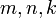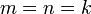# Beal conjecture

## History

This conjecture was made by Andrew Beal, a mathematics hobbyist, while investigating Fermat's last theorem.

## Statement

Consider the equation:.

The Beal conjecture (also called Beal's conjecture) states the following equivalent things:

1. This equation has no solutions forpairwise relatively prime positive integers, andall natural numbers greater than.
2. This equation has no solutions forpairwise relatively prime integers (all nonzero) andall natural numbers greater than.

## Related facts

### Weaker facts and conjectures

• Fermat's last theorem: This is the special case. This was conjectured by Fermat and proved by Wiles, building on work by several mathematicians in between.